Smartick is an online platform for children to master math in only 15 minutes a day

Oct14

# What Is an Angle? How Is It Made?

In today’s post we are going to answer the question, what is an angle?

To begin, it would be a good idea for you to review the following link where we have explained the concept of ”straight lines.”

Now that you remember straight lines, let’s begin!

In order to understand what an angle is, we are going to construct one.

We start by drawing a ray on the plane: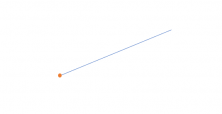But we cannot construct an angle with just one ray, we need two.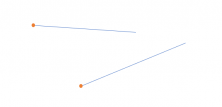Now, even though we have two rays we still do not have an angle because our rays need specific characteristics. Do you know what they are?

To construct an angle the two rays need to leave from the same point, known as a VERTEX.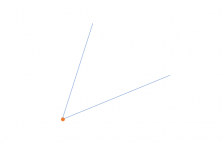Now we can see it because an angle is the portion of the plane that is between the two rays and their vertex.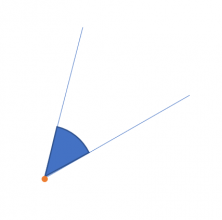But look closely! Two rays from the same origin do not just form one angle, but two!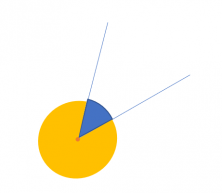An interior (blue) and an exterior angle (yellow).

If the lines crossed instead of originating from the same place, do you know how many angles they would form? If you know the answer, leave it in the comments!

Now you know what an angle is and how to construct one. If you want to know more about them, you can look at the following links to learn about the different types there are according to their names, their measurements, exercises and much more.

I hope that this information has helped to answer any questions you may have had. To learn and practice geometry and elementary mathematics adapted to your level, register with Smartick and start a free trial.